# How to Use a Pilot Watch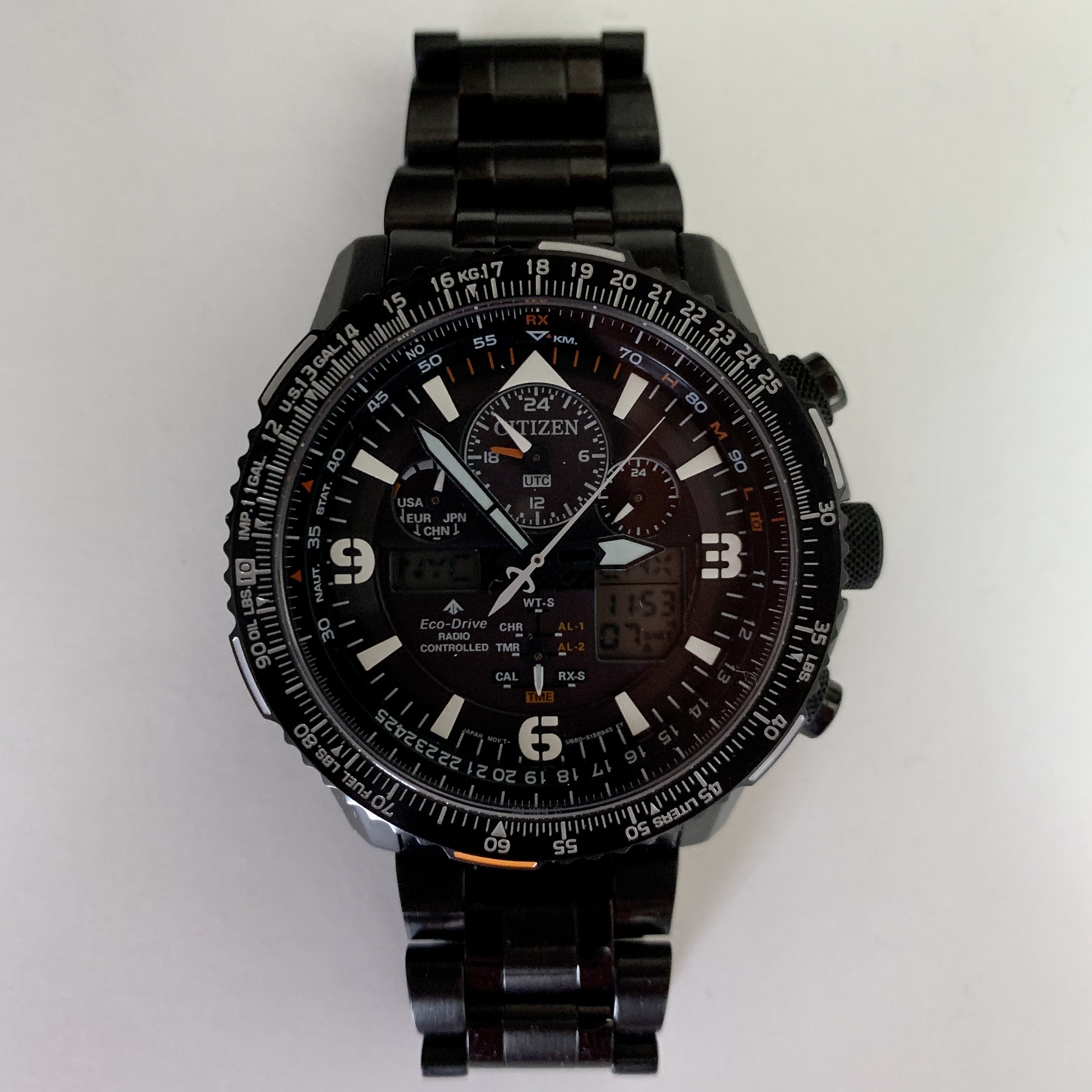This is a brief tutorial for performing basic computations using the E6B flight computer on a pilot watch.

# What is an E6B?

An E6B flight computer is a circular slide rule used by pilots to make common aviation calculations. A pilot watch typically contains a simplified version of a full E6B like the one shown below.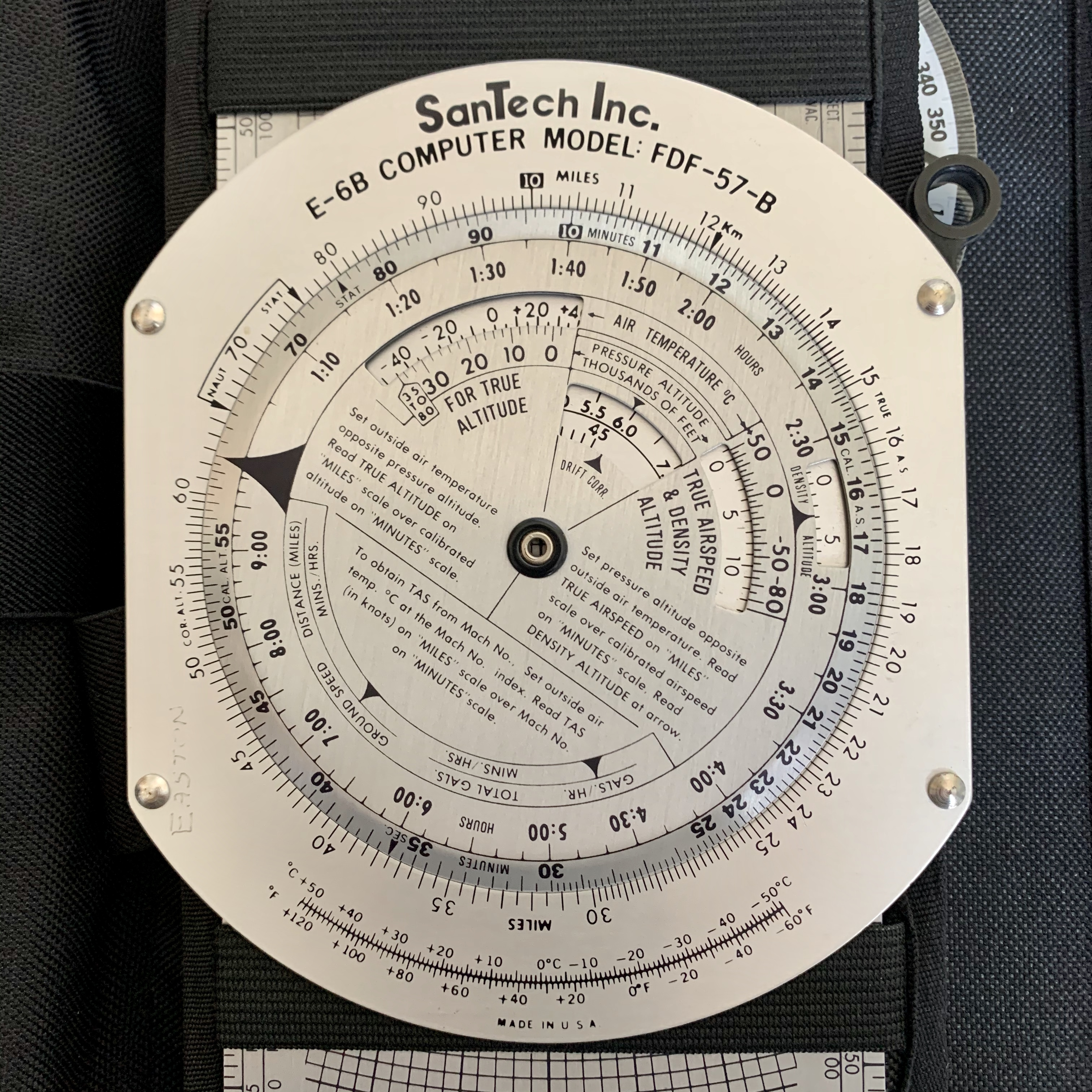A US Navy officer developed the E6B—or "whiz wheel"—in the 1930s and it was first used on a large scale in WWII1. The design is pretty much unchanged since then and every student pilot learns how to make basic calculations for flight planning using a standard E6B. It found its way onto a watch with the Navitimer pilot watch introduced by Breitling in the 1950s2, and we will use the popular Citizen Skyhawk (manual) for these examples.

# Examples

The pilot watch, like a standard E6B, consists of two concentric scales, one of which rotates. Notice that both have special markings for the 10 position. We will reference one or the other of these frequently to identify the solution opposite them.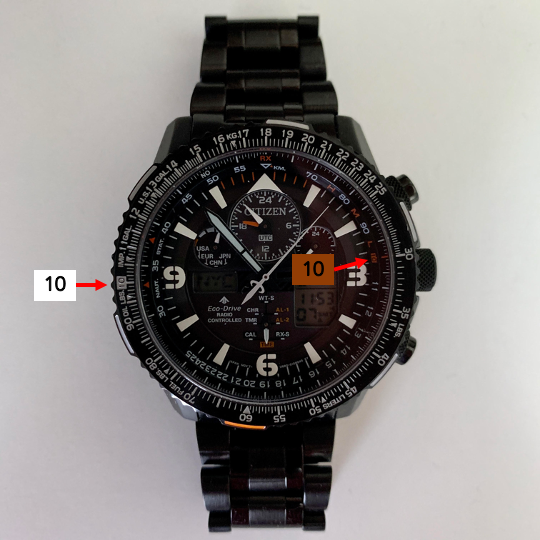## Division

The most common computations on a pilot watch involve multiplication and division. We will start with division since it is the most straightforward: simply rotate the bezel so the two numbers to divide are over each other and read the result opposite the 10.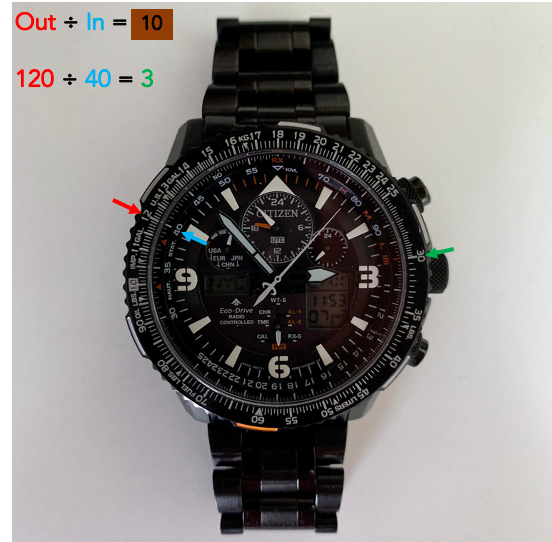## Multiplication

Since division is easy to remember, the multiplication method I prefer is the exact opposite. Set the first number to multiply opposite the red 10, go to the second number to multiply on the inner bezel, and read the answer opposite it.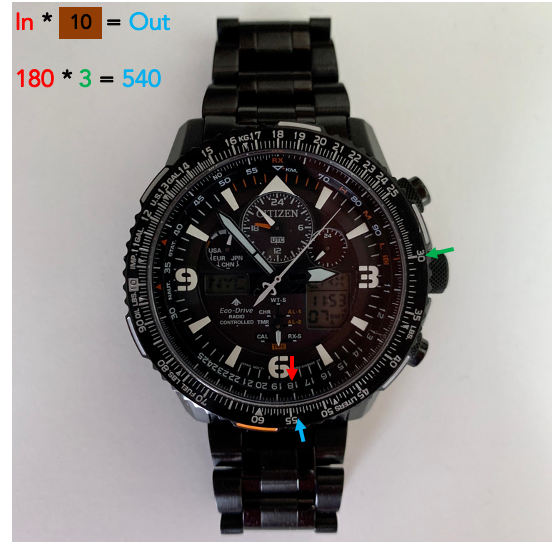An alternative method is to rotate the white 10 opposite the first number, find the second number on the outer bezel, and read the result on the inner bezel opposite from it.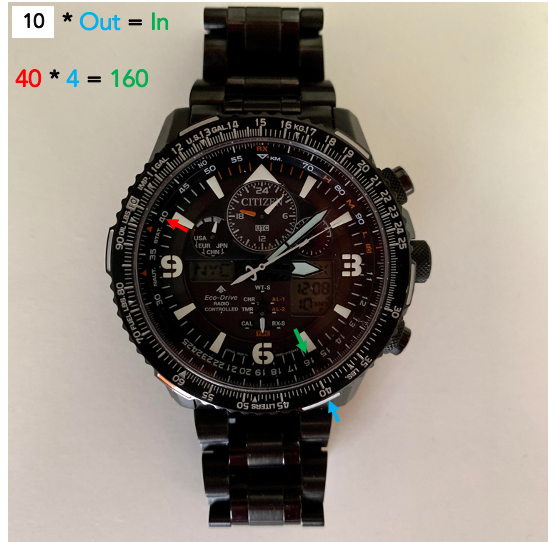Here is another multiplication example: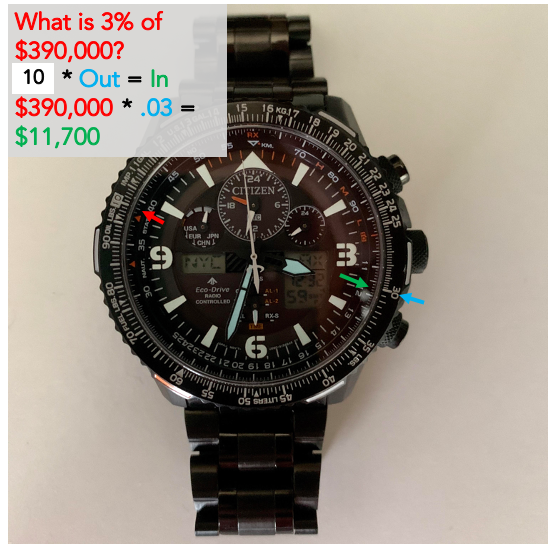For a final practical example, say you need to split a $77 bill between 3 people and want to include a 20% tip, how much does each person pay? First divide 77 by 3 to determine that each person owes about$25.50 before the tip. Moving from 10 up to 12 (or 1.2), we see that with the tip each person owes about \$31.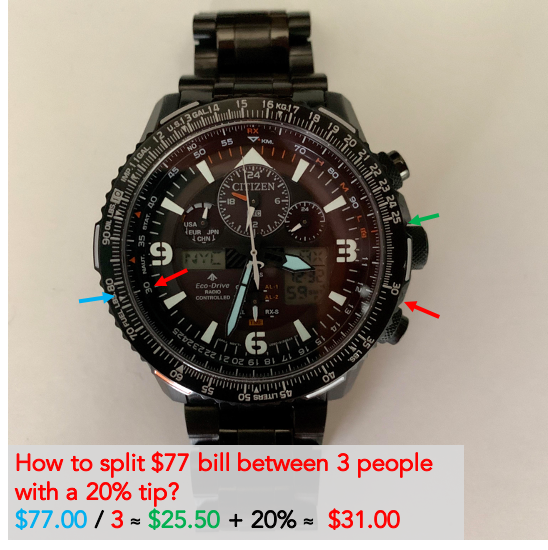Inevitably I will forget exactly how to set the bezels and whether to read off the inside or outside one. To remind myself, I pick a simple relationship that I know the answer to and use that pattern to do the multiplication or division I can't do in my head.

## Conversions

The pilot watch has markings for several conversions including distance and fuel units. For example, it is easy to convert distance between nautical miles, statute miles, and kilometers.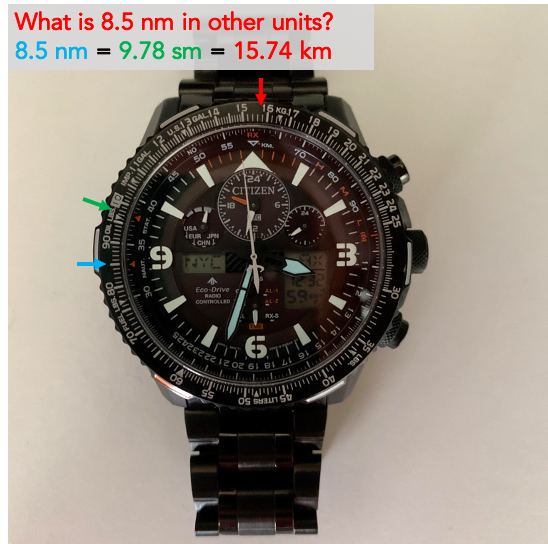You can also convert between pounds and kilograms: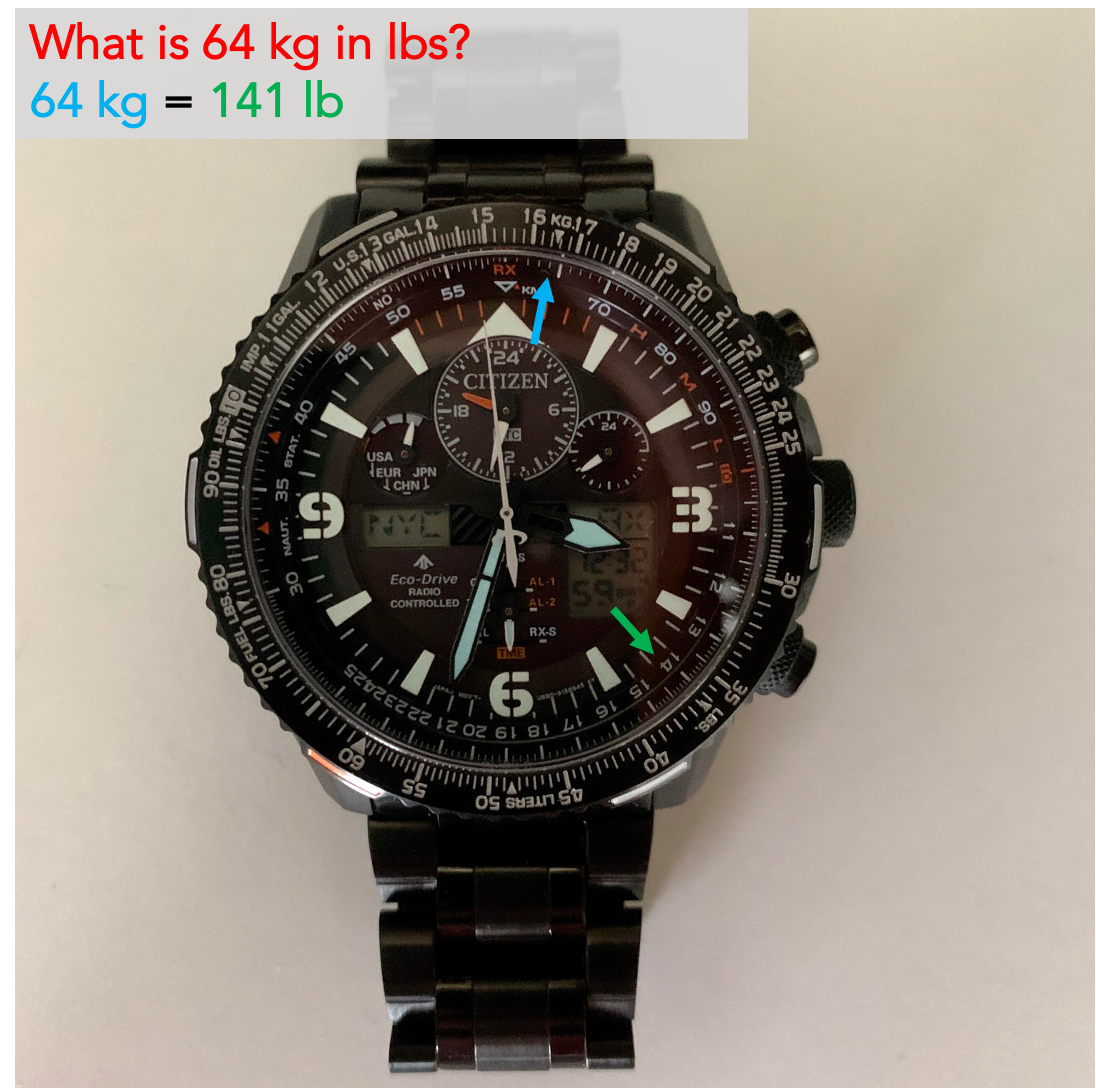## Distance, Rate, and Time

Next we will demonstrate how to solve for distance, rate, or time given the other two variables. These are given by the following relationship:

Distance [unit] = Rate [unit/time] * Time [time]


If the time units are the same, you can use the multiplication and division examples above with the 10 index. However, if one is in hours and one is in minutes, we instead reference the below relationships:

Distance [unit] = Rate [unit/hr]      * Time [min] * 1/60 [hr/min]
Distance [nm]   = Rate [knot = nm/hr] * Time [min] * 1/60 [hr/min]
Distance [gal]  = Rate [gal/hr]       * Time [min] * 1/60 [hr/min]


To save from needed to divide or multiply by 60 to convert between hours and minutes, we instead use 60 or MPH at the 12 o'clock position as the index rather than the 10.

Distance (or amount, like gallons) is show on the outer bezel, time in minutes on the inner bezel, and the rate in distance per hour at the 60 position. I remember this as follows:

• Rate at the top
• Time in minutes on the inner bezel (closest to the hands of the watch that tell time)
• Distance on the outer bezel (which also has markings for distance and fuel conversions)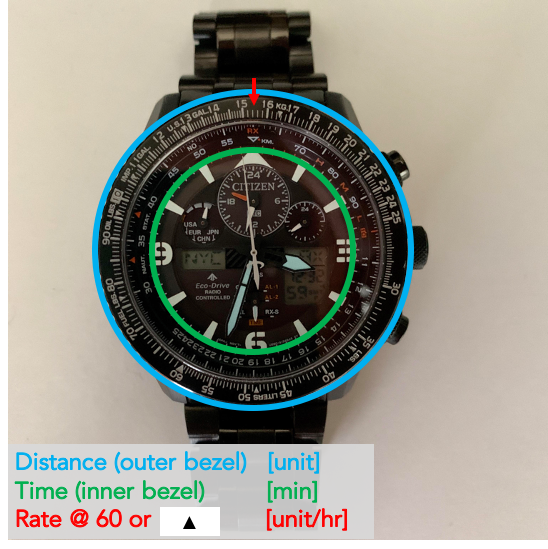First, let's calculate rate—or airspeed—given distance and time. Rotate the bezel so the distance on the outer ring is over the time on the inner bezel, and read the rate across from the 60 at the top of the watch.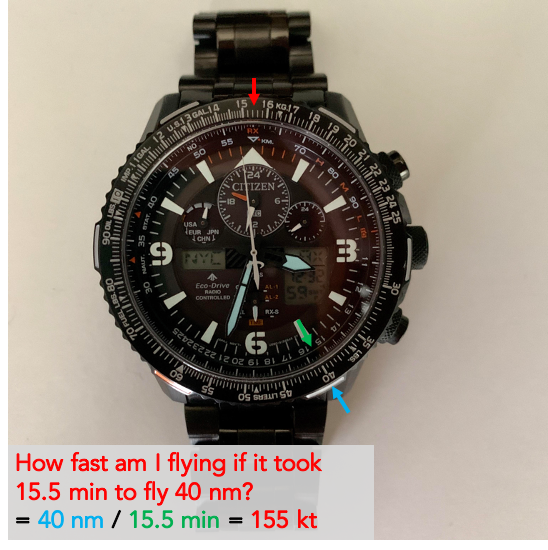To calculate time (in minutes) given speed and distance, set your speed at the top position and read the time on the inner bezel across from the distance on the outer bezel. Note in this example that the distance units don't matter as long as they are consistent. Here they are mph and miles, but they could also be knots and nm, gal/hr and gallons, etc.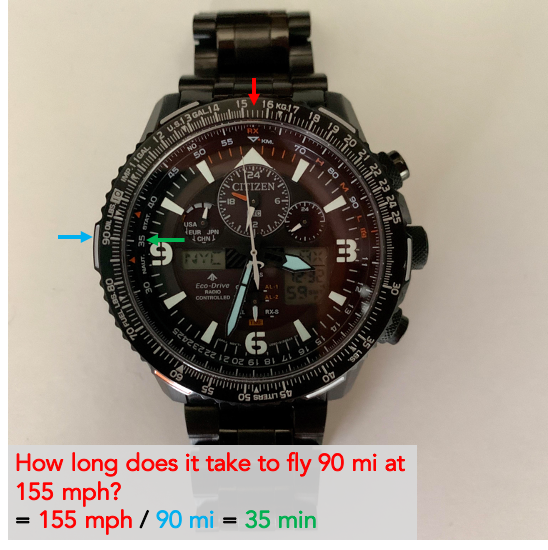Likewise, to calculate distance given speed and time, set your speed at the top position and read the distance on the outer bezel across fro mthe time on the inner bezel.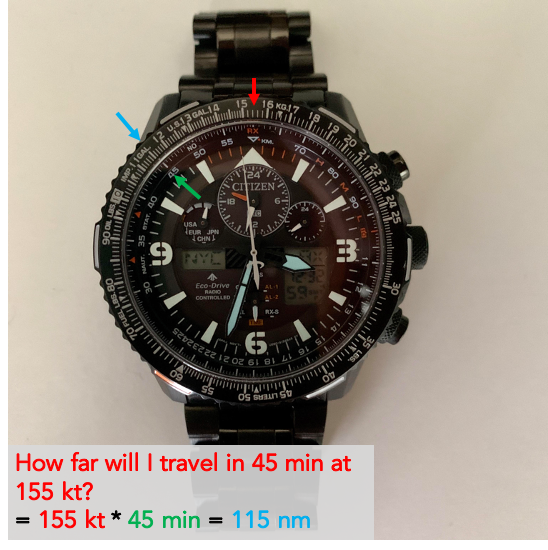As mentioned above, we can substitue other "distance" units, in this case gallons per hour and gallons rather than airspeed and distance.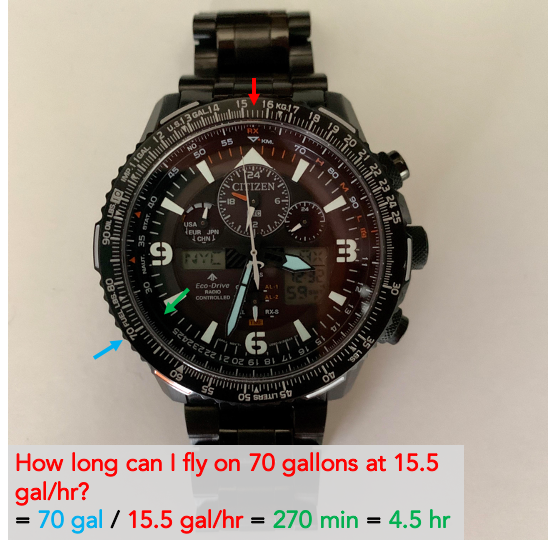In this final example we calculate the distance from an airport to start a descent in two steps.

1. First, divide the altitude to descend by the desired descent rate to calculate the time to descend.
2. Next, determine your speed in nm/min by dividing your speed in knots (nm/hr) by 60 either using the watch or mentally.
3. Finally, multiply the time to descend by your groundspeed to calculate the distance from the airport to start the descent.

If you are able to complete step 2 in your head, you can get straight to the answer with the outer bezel set in one position as show below.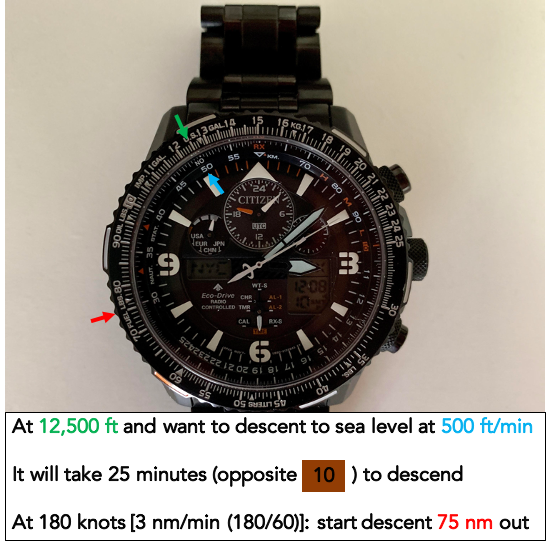# Resources

Here are some videos I found helpful that include additional examples for how to use a pilot watch:

1. Valerio, Pablo. “E6B Computer: Celebrating 75 Years Of Flight.” InformationWeek, Information Week, 28 Dec. 2015, www.informationweek.com/government/e6b-computer-celebrating-75-years-of-flight/a/d-id/1323695

2. “Pilot Chronograph Watch Functions & How to Use a Chronograph Watch?” WatchGecko, 27 Feb. 2017, www.watchgecko.com/pilot-chronograph-watch-functions/

© 2005 Matthew Kudija | Source